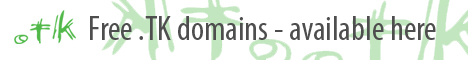.tk Renaming Internet### Permute the Last Layer (PLL)

The PLL is the final step in most methods. This is where you will switch pre-oriented pieces to their final solved positions without affecting orientation. Since we are preserving orientation, these algorithms are generally much longer than OLL algorithms. Below is a Full list of PLL algorithms; there are 21 cases. Most speedcubers will never learn all of these, but everyone should know a select few. Beginners should know the algorithms with orange names. Ambitious beginners might try learning some blue ones as well! Intermediates should also know algorithms with sky blue names, and make it a goal to know them all one day! Advanced solvers must know all of them, and be comfortable with performing them quickly! Remember that these are not the only algorithms for each case, just my favorites. If you dislike an algorithm, find another one from a different website.

The PLL images on this page were made created by me. I thought it would be nice to have the traditional arrows, but also have a color recognition in there too. It might help you to see where certain blocks of completed pieces are, rather than just arrows. For the G Series, I didn't use arrows at all. If you don't know why, go look at someone elses G perm images; they are a goddamn mess, and nearly indecipherable. G permutation is actually very easily recognizable by placing the headlights left and then looking for the 1x1x2 block in one of four possible places.

**If you wish to use these PLL images, you will require my permission. Please send me an email with your website URL.Ua PermutationR2 U R U R' U' R' U' R' U R' y2 R' U R U' R' U' R' U R U R2Ub PermutationR U' R U R U R U' R' U' R2y2 R2 U' R' U' R U R U R U' RH PermutationM2 U M2 U2 M2 U M2Z PermutationU R' U' R U'R U R U' R' U R U R2 U' R' UU' M' U M2 U M2 U M U2 M2Aa Permutationx' R' D R' U2 R D' R' U2 R2Ab Permutationx' R2 U2 R D R' U2 R D' RE Permutationx' R U' R' D R U R' u2 R' U R D R' U' RJ PermutationR U R' F' R U R' U' R' F R2 U' R' U'L PermutationL U' R' U L' U2 R U' R' U2 RF Permutationy R' U2 R' U' y R' F' R2 U' R' U R' F R U' F(yeah, yeah, I'll rotate the image later.)V Permutation R' U R' U' y R' F' R2 U' R' U R' F R FT Permutation R U R' U' R' F R2 U' R' U' R U R' F'Y Permutation F R U' R' U' R U R' F' R U R' U' R' F R F'Ra PermutationR U R' F' R U2' R' U2 R' F R U R U2' R' U'Rb Permutation R' U2 R U2 R' F R U R' U' R' F' R2 U'Ga PermutationR2 u R' U R' U' R u' R2 (F' z) R bGb PermutationR' d' F R2 u R' U R U' R u' R2Gc Permutation R U R' y' R2 u' R U' R' U R' u R2Gd PermutationR2 u' R U' R U R' u R2 f R' f'Na Permutation R' U R U' R' F' U' F R U R' F R' F' R U' RNb PermutationR U' R' U l U F U' R' F' R U' R U l' U R'

### 2-look PLL: (Intermediate)

A 2-Look PLL is never more than two steps long, and very consistent with how fast it can be performed... which is very, very important for speedsolvers! So, how do you do a 2-Look PLL?

Well, first, you need to learn the first seven PLL algorithms listed in the section above. Then, you need to practice them a lot until they are fast and reliable (Under 4 seconds each might be a good rule of thumb). Lastly, learn to permute your corners first in just one algorithm (Aa, Ab, or E), and your edges second in just one algorithm (Ua, Ub, H, or Z).

After you master this method, your PLL should give you no problems, and will be very reliable and fast. After you're good at it and ready to learn more, start working on learning more PLLs -- essentially 1-step shortcuts.

Most serious speedcubers will end up learning all of the PLLs over the course of a few months, but it's wise to learn how to do a 2-Look first, and get fast at it before you start learning more. Learn them one at a time, and don't worry about learning them all quickly (it took me most of a year)! Just practice a lot.. a fast PLL is not what makes a fast speedcuber.

Confuzzled? Here's my YouTube video explaining the whole thing:

2-look PLL Tutorial (Intermediate)

Want to see more? Here's my channel: www.youtube.com/user/lancetheblueknight

Katekyo Hitman Reborn